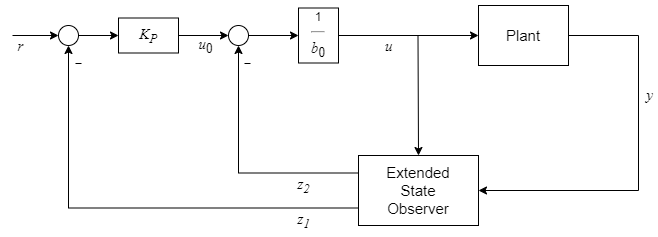## Active Disturbance Rejection Control

Active disturbance rejection control (ADRC) is a model-free control technique that is useful for designing controllers for plants with unknown dynamics and internal and external disturbances. This algorithm requires only an approximation of the plant dynamics to design controllers that provide robust disturbance rejection with no overshoot.

You can use the Active Disturbance Rejection Control block to implement ADRC. The block uses a first-order or second-order model approximation of the known system dynamics along with the unknown dynamics and disturbances modeled as an extended state of the plant. Typically, you determine this order from the open-loop step response of your plant in the operating range.

• First-order approximation — $\stackrel{˙}{y}\left(t\right)={b}_{0}u\left(t\right)+f\left(t\right)$

• Second-order approximation — $\stackrel{¨}{y}\left(t\right)={b}_{0}u\left(t\right)+f\left(t\right)$

Here:

• y(t) is the plant output.

• u(t) is the input signal.

• b0 is the critical gain, which is the estimated gain that describes the plant response to an input u(t).

• f(t) is the total disturbance, which includes unknown dynamics and other disturbances.

The block uses an extended state observer (ESO) to estimate f(t) and implements disturbance rejection control by reducing the effect of estimated disturbances on the known part of model approximation.

You can implement both discrete-time and continuous-time controllers. Set the controller time domain and sample time to match the time domain of the plant model.

### Controller Structure

#### First-Order Approximation

For a first-order plant model approximation $\stackrel{˙}{y}\left(t\right)={b}_{0}u\left(t\right)+f\left(t\right)$, the plant output state is x1 = y(t) and the extended state is x2 = f(t).

The state space model is as follows.

`$\begin{array}{l}\stackrel{˙}{x}=Ax+Bu+\left(\begin{array}{c}0\\ \stackrel{˙}{f}\left(t\right)\end{array}\right)\\ y=Cx,\end{array}$`

where

`$A=\left(\begin{array}{cc}0& 1\\ 0& 0\end{array}\right),B=\left(\begin{array}{c}{b}_{0}\\ 0\end{array}\right),C=\left(\begin{array}{cc}1& 0\end{array}\right).$`

For this observable system, the block uses a Luenberger observer to provide an estimate of the plant states and total disturbances. Using the estimated states

`$\begin{array}{l}{z}_{1}=\stackrel{^}{y}\left(t\right)\\ {z}_{2}=\stackrel{^}{f}\left(t\right),\end{array}$`

the controller computes the control input u(t) as follows.

`$u\left(t\right)=\frac{{u}_{0}\left(t\right)-{z}_{2}}{{b}_{0}},$`

where

`${u}_{0}\left(t\right)={K}_{P}\left(r\left(t\right)-{z}_{1}\right).$`

This is an estimation-based state feedback controller, and when the estimated and actual values are equal, the system has a first-order closed-loop behavior. This closed loop system has the pole s = –KP.

This controller is represented as the following control structure.For controller tuning simplicity, the block sets the controller pole at (s + ωc) and the observer poles at (s + ωo)2, where ωc and ωo are the controller and observer bandwidths, respectively.

#### Second-Order Approximation

For a second-order plant model approximation $\stackrel{¨}{y}\left(t\right)={b}_{0}u\left(t\right)+f\left(t\right)$, the plant output states are x1 = y(t) and x2 = $\stackrel{˙}{y}\left(t\right)$, and the extended state is x3 = f(t).

The state space model is as follows.

`$\begin{array}{l}\stackrel{˙}{x}=Ax+Bu+\left(\begin{array}{c}0\\ 0\\ \stackrel{˙}{f}\left(t\right)\end{array}\right)\\ y=Cx,\end{array}$`

where

`$A=\left(\begin{array}{ccc}0& 1& 0\\ 0& 0& 1\\ 0& 0& 0\end{array}\right),B=\left(\begin{array}{c}0\\ {b}_{0}\\ 0\end{array}\right),C=\left(\begin{array}{ccc}1& 0& 0\end{array}\right).$`

For this observable system, the block uses a Luenberger observer to provide an estimate of the plant states and total disturbances. Using the estimated states

`$\begin{array}{l}{z}_{1}=\stackrel{^}{y}\left(t\right)\\ {z}_{2}=\stackrel{^}{\stackrel{˙}{y}}\left(t\right)\\ {z}_{3}=\stackrel{^}{f}\left(t\right),\end{array}$`

the controller computes the control input u(t) as follows.

`$u\left(t\right)=\frac{{u}_{0}\left(t\right)-{z}_{3}}{{b}_{0}},$`

where

`${u}_{0}\left(t\right)={K}_{P}\left(r\left(t\right)-{z}_{1}\right)-{K}_{D}{z}_{2}.$`

This is an estimation-based state feedback controller, and when the estimated and actual values are equal, the system has a second-order closed-loop behavior.

This controller is represented as the following control structure.For controller tuning simplicity, the block sets the controller poles at (s + ωc)2 and the observer poles at (s + ωo)3, where ωc and ωo are the controller and observer bandwidths, respectively.

### Specify Controller Parameters

To implement ADRC for your plant, it is essential you provide a reasonable guess of the critical gain value b0 for the plant approximation. One method of doing so is as follows.

1. Simulate the plant in open loop over the operating range using a step signal with magnitude uOL.

2. Record the change in plant output over a short duration of time.

• For first-order ADRC, use the response approximation y = at and determine a as follows.

`$a=\frac{y\left(end\right)-y\left(0\right)}{t\left(end\right)-t\left(0\right)}$`

For an example, see Design Active Disturbance Rejection Control for Water-Tank System.

• For second-order ADRC, use the response approximation y = ½at2 and determine a as follows.

`$a=\frac{2\left(y\left(end\right)-y\left(0\right)\right)}{{\left(t\left(end\right)-t\left(0\right)\right)}^{2}}$`

For an example, see Design Active Disturbance Rejection Control for Boost Converter.

3. You can then determine b0 from a and the step magnitude uOL.

`${b}_{0}=\frac{a}{{u}_{OL}}$`

Use the Critical gain parameter to set this value in the block parameters.

Then, to tune the controller response, specify the ωc and ωo values using the Controller bandwidth and Observer bandwidth, respectively. These values depend on the performance requirements of your controller. In general, a faster response requires a larger controller bandwidth. The observer also needs to converge faster than the controller. Therefore, set the observer bandwidth to 5 to 10 times the controller bandwidth.

Additionally, the block allows you to specify initial conditions for states, limit controller output, and output estimated state values.

 Herbst, Gernot. “A Simulative Study on Active Disturbance Rejection Control (ADRC) as a Control Tool for Practitioners.” Electronics 2, no. 4 (August 15, 2013): 246–79. https://doi.org/10.3390/electronics2030246.

 Zhiqiang Gao. “Scaling and Bandwidth-Parameterization Based Controller Tuning.” In Proceedings of the 2003 American Control Conference, 2003, 6:4989–96. Denver, CO, USA: IEEE, 2003. https://doi.org/10.1109/ACC.2003.1242516.